# ISEE Middle Level Math : Data Analysis

## Example Questions

### Example Question #9 : How To Find Range

Find the range in this set of numbers:

9080, 9008, 9800, 9099, 9009, 9090, 9008

Possible Answers:Correct answer:Explanation:

First, order the numbers from least to greatest: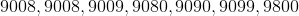The, subtract the smallest number from the greatest: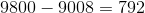Answer: The range is 792.

### Example Question #10 : How To Find Range

Find the range in this set of numbers: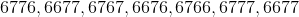Possible Answers:Correct answer:Explanation:

First, order the numbers from least to greatest: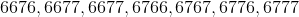Then, find the difference between the largest and smallest number: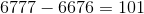Answer: The range is 101.

### Example Question #11 : Data Analysis And Probability

Find the range in this set of numbers:

5855, 5588, 5585, 5858, 5885, 5558, 5885

Possible Answers: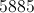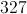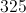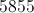Correct answer:Explanation:

First, order the numbers from least to greatest: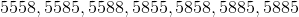Then, find the difference between the largest and smallest number: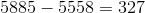Answer: The range is 327.

### Example Question #11 : How To Find Range

Find the range in this set of numbers:

212, 112, 121, 221, 122

Possible Answers: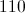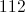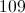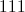Correct answer:Explanation:

First, order the numbers from least to greatest: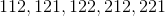Then, subtract the smallest number from the largest: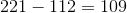Answer: The range is 109.

### Example Question #11 : Range

Find the difference between the mode and the range in this set of numbers:

9881, 9981, 9818, 9981, 9891, 9918, 9989, 9889, 9198

Possible Answers: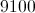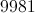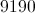Correct answer:Explanation:

First, order the numbers from least to greatest: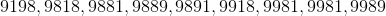Then, identify the mode (the most recurring number in the set):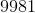Then, find the range (difference of the largest and smallest number):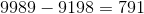Finally, find the difference between the mode and the range in this set: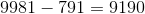### Example Question #14 : Data Analysis And Probability

Find the range in this set of numbers: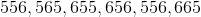Possible Answers: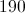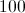Correct answer:Explanation:

First order the numbers from least to greatest: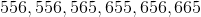Then, subtract the smallest number from the largest number: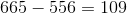Answer: The range is 109.

### Example Question #15 : Data Analysis And Probability

Find the range in this set of numbers:

787, 778, 878, 877

Possible Answers: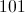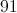Correct answer:Explanation:

First, order the numbers from least to greatest: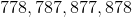Then, subtract the smallest number from the largest number: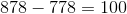Answer : The range is 100.

### Example Question #321 : Isee Middle Level (Grades 7 8) Mathematics Achievement

Find the range of this set of numbers: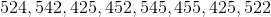Possible Answers: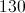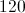Correct answer:Explanation:

The range is the difference between the smallest and largest numbers.

First, order the numbers from least to greatest: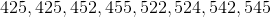Then subtract the smallest from the largest number: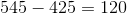### Example Question #11 : Data Analysis And Probability

Find the range of this set of numbers: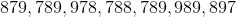Possible Answers: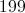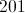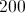Correct answer:Explanation:

The range is the difference between the smallest and largest number.

First, order the numbers from least to greatest: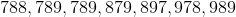Then, subtract the smallest from the largest number: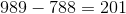### Example Question #11 : Data Analysis

Find the range of this set of numbers: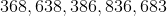Possible Answers: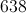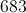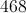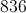Correct answer:Explanation:

The range of a data set is the difference between the smallest and largest numbers.

First, order the numbers from least to greatest: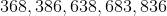Then, subtract the smallest number from the largest: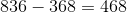### All ISEE Middle Level Math Resources